Ex 12.3

Chapter 12 Class 10 Areas related to Circles
Serial order wise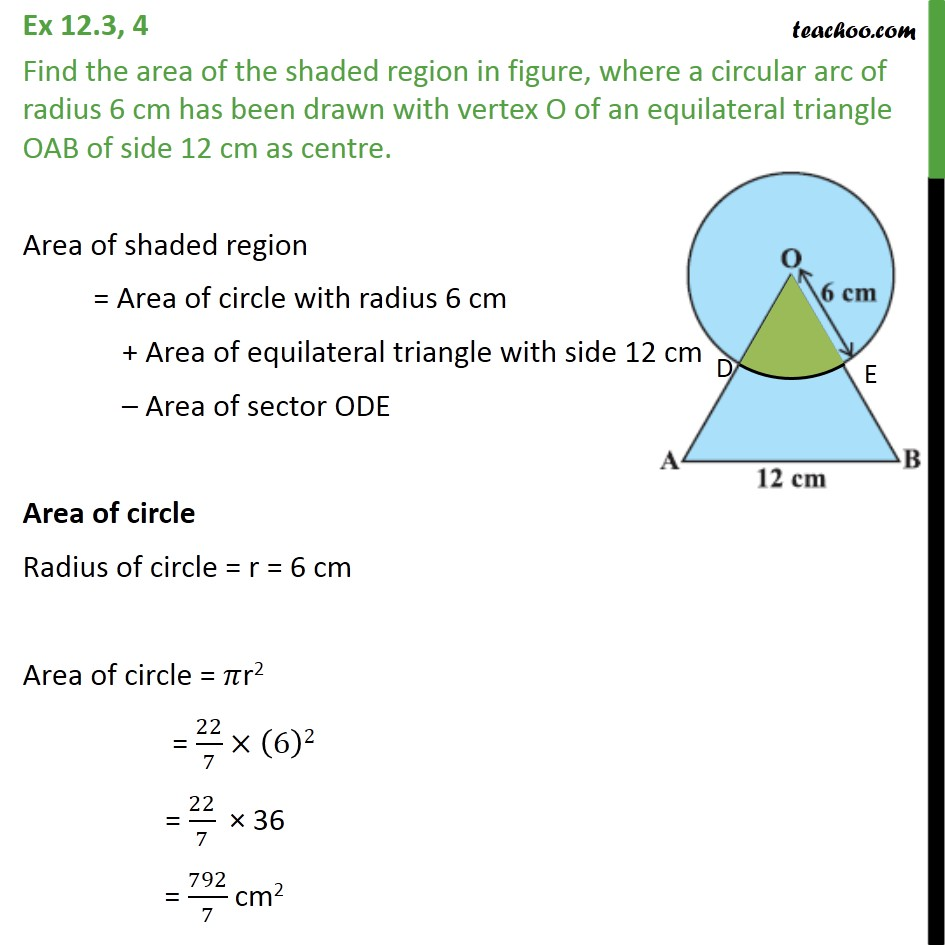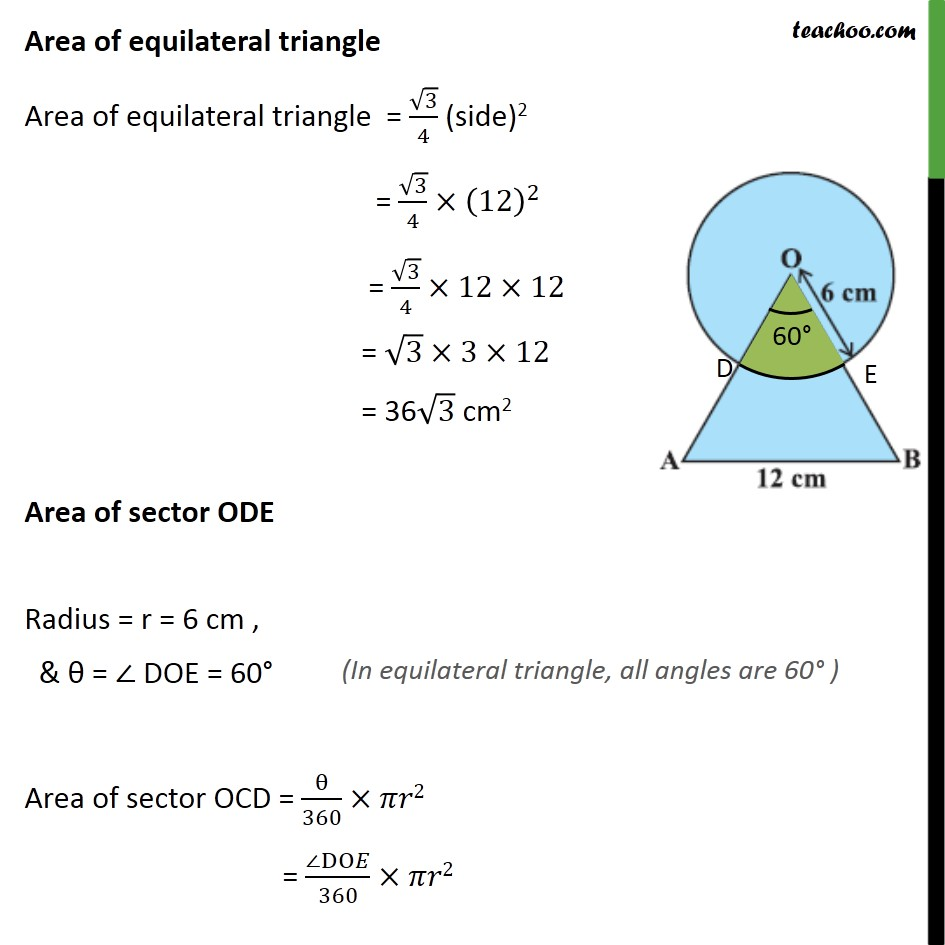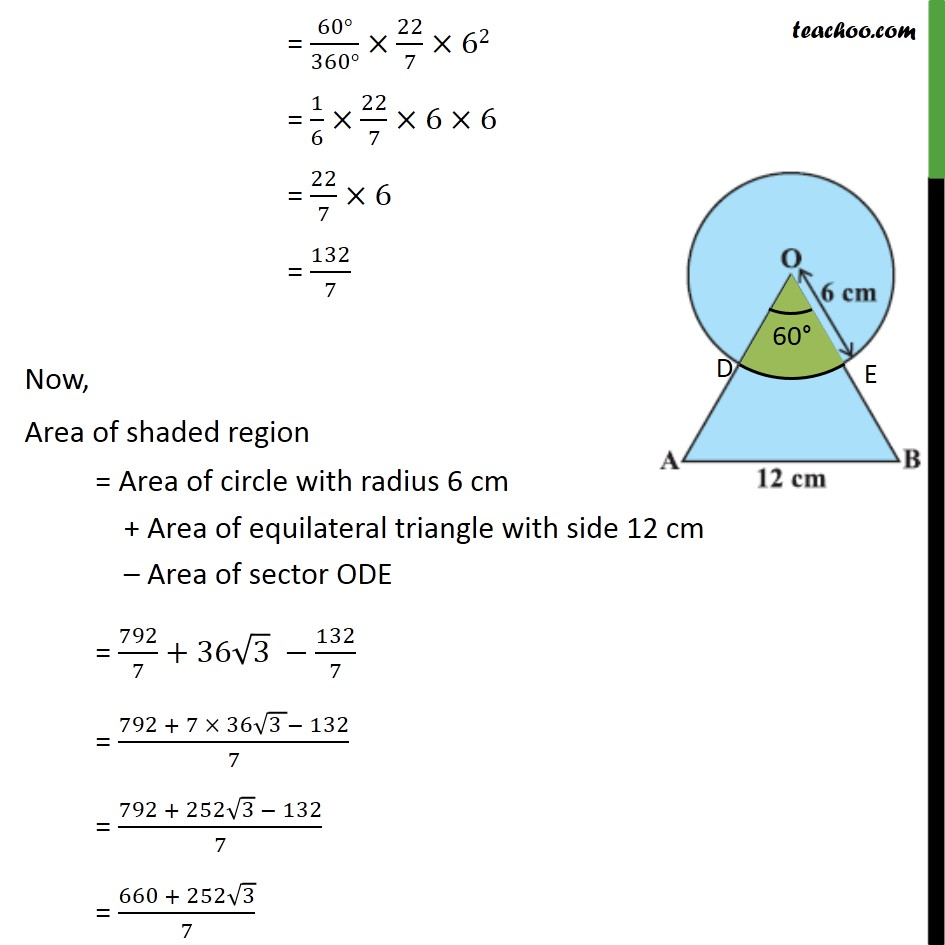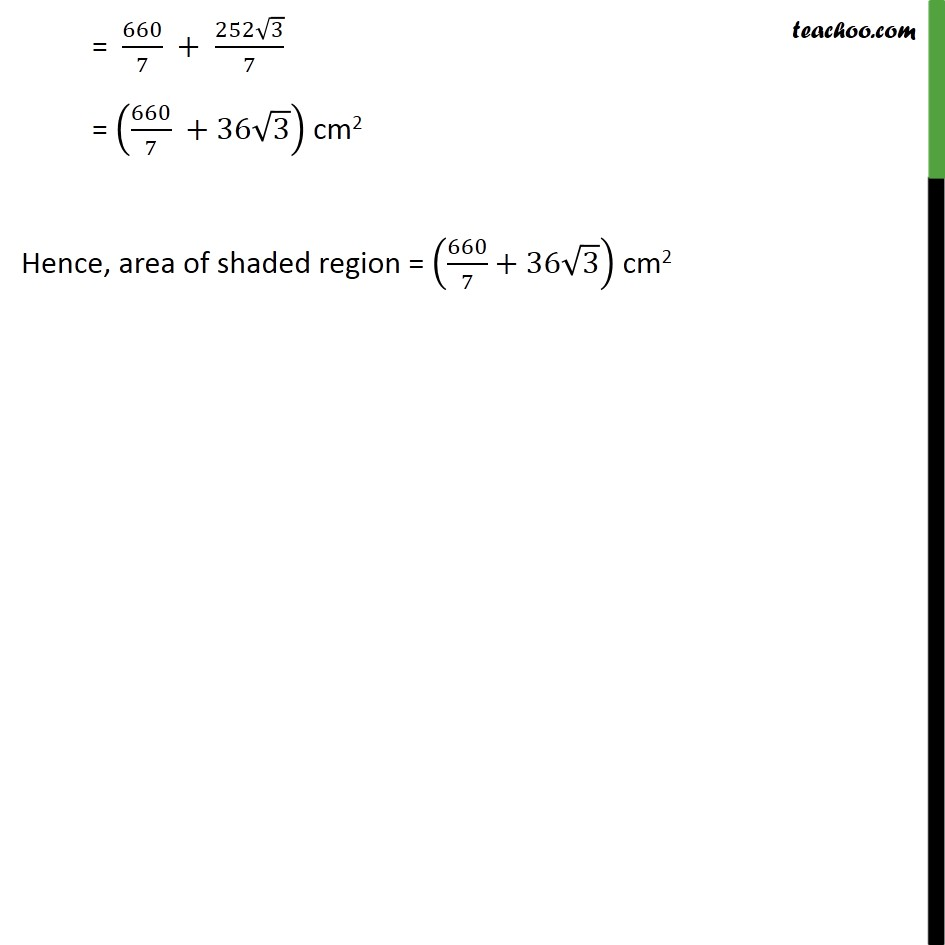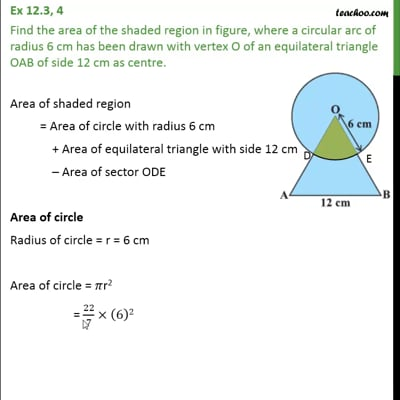This video is only available for Teachoo black users

Solve all your doubts with Teachoo Black (new monthly pack available now!)

### Transcript

Ex 12.3, 4 Find the area of the shaded region in figure, where a circular arc of radius 6 cm has been drawn with vertex O of an equilateral triangle OAB of side 12 cm as centre. Area of shaded region = Area of circle with radius 6 cm + Area of equilateral triangle with side 12 cm – Area of sector ODE Area of circle Radius of circle = r = 6 cm Area of circle = 𝜋r2 = 22/7×(6)2 = 22/7 × 36 = 792/7 cm2 Area of equilateral triangle Area of equilateral triangle = √3/4 (side)2 = √3/4×(12)^2 = √3/4×12×12 = √3×3×12 = 36√3 cm2 Area of sector ODE Radius = r = 6 cm , & θ = ∠ DOE = 60° Area of sector OCD = θ/360×𝜋𝑟2 = (∠DO𝐸)/360×𝜋𝑟2 = (60°)/(360°)×22/7×62 = 1/6×22/7×6×6 = 22/7×6 = 132/7 Now, Area of shaded region = Area of circle with radius 6 cm + Area of equilateral triangle with side 12 cm – Area of sector ODE = 792/7+36√3 −132/7 = (792 + 7 × 36√(3 )− 132)/7 = (792 + 252√3 − 132)/7 = (660 + 252√3)/7 = 660/7 + (252√3)/7 = (660/7 +36√3) cm2 Hence, area of shaded region = (660/7+36√3) cm2